Modal Analysis

Since every structure (machine, building, car, bicycle, home electric appliance, etc.) has a natural frequency, it is necessary to know the natural frequency and how it vibrates at frequencies other than the natural frequency. Modal analysis is software which simulates the conditions when vibrations of each frequency are applied to various structures. At present, modal analysis can be performed easily using a personal computer or the like based on the transfer characteristics on each structure obtained using the FFT analyzer together with a shaker and a vibration pickup. This makes it possible to find structural weak points and take effective vibration and noise insulation measures.

Nichols Diagram

The Nichols diagram displays the transfer function using the horizontal axis as phase and the vertical axis as gain

Nyquist Diagram

The Nyquist diagram indicates the frequency characteristics with the real part and the imaginary part of the frequency response function assigned to the horizontal axis and vertical axis, respectively. It is used mainly to determine the stability of control systems.

Octave Analysis

The power spectrum represents the power for each band by splitting the frequency into a fixed width. Conversely, with an analog frequency analyzer with the frequency axis assigned the logarithm scale, frequency analysis is performed by passing the signal through a constant-width band-pass filter which splits the axis at equal intervals. Generally, the bandwidth is 1-octave and 1/3-octave. This kind of analysis is referred to as octave analysis, and is used widely in the field of sound measurement.

Generally, when the upper cut-off frequency, ?, is twice the lower cut-off frequency, f1, the interval between f1 and ? is an octave. The center frequency of the octave is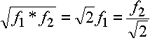1/3 octave refers to an octave divided by three. With 3/1 octave, f2 is 21/3 times f1 and the center frequency is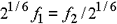The ANSI CLASS III standard specifies the center frequency of the octave band and filter characteristics. Analog octave analyzers are unified to this standard.

Open-Loop/Closed-Loop Operation

The measured open-loop or closed-loop transfer function can be used to give a closed-loop or open-loop transfer function, respectively.

If there is no feedback element, the obtained open-loop transfer function GO gives the following closed-loop transfer function GC: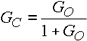The obtained closed-loop transfer function GC gives the following open-loop transfer function GO: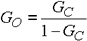Orbit

A figure drawn by synthesizing two signals applied to orthogonal X and Y axes is referred to as a Lissajous figure, or "Orbit."  It indicates visual characteristics of two signals with the combination of the amplitude, frequency ratio, and phase difference of these signals. When the frequency of one signal is an integer multiple of the other, the figure returns to the starting point in a constant period.

Overall Value

The overall value is the total (overall) power of the frequency range under analysis.

The overall value can be obtained by the following expressions.

1. When the reference value is single-ended value (Peak value) (Ono Sokki Model: CF-350/360, CF-900 Series, CF-880, etc.)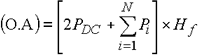1. When the reference value is the RMS value (Ono Sokki Model: CF-5000 Series, CF-3000 Series, DS-2000 Series)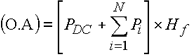where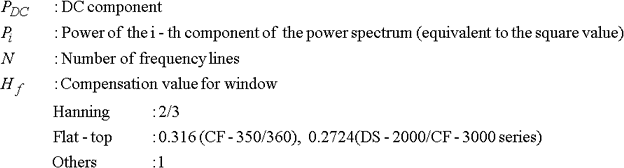* The value of Hf depends on the model.  Refer to your instruction manual for details.

PDC and Pi are power (Single-ended value)2 and (RMS value)2, respectively.

Partial overall is the total power within the range of the specified frequency section.

Overlap Processing

In the case of the real-time analysis HREF_REALTIME frequency or lower, the window can be overlapped to perform FFT analysis.

Take an example where a FFT operation is performed for data every 1,024 points. At this time, FFT analysis is performed by overlapping the newly sampled data with the previous data. A larger amount of overlap means tracking of signal variation at high speed.

Phase Spectrum

There are two modes of phase display as a frequency function:

(1) the phase spectrum of one channel
(2) the phase difference between two channels.

(1) Phase spectrum of one channel

The Fourier transform of time function x (t) is given by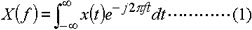X (f) in expression (1) is especially referred to as the (complex) Fourier spectrum. Since X (f) is a complex function, it can be represented as amplitude |X (f)| and phase θ (f) using the real part, XR (f), and the imaginary part, XI (f).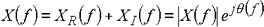Therefore,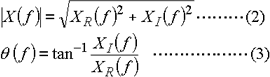Expression (2) is the amplitude of the Fourier spectrum (MAG display). With this analyzer, expression (3) is referred to as the phase spectrum.

With this analyzer, the starting point of a frame is the origin and the phase of the cosine wave is assumed to be 0 degrees. Even if X (f) in expression (2) is the same, the waveform of time signal x (t) differs largely if phase spectrum θ (f) is different.

When measuring the phase spectrum, the trigger function is usually used to measure the phase spectrum with respect to a certain time. As an application, the phase spectrum is used for field balancing of a body of rotation.

(2) Phase difference between two channels

The phase difference between two channels is obtained as a phase spectrum of the transfer function or cross spectrum which is a complex function. If the transfer function of the system (frequency response function) is H (f), it is represented by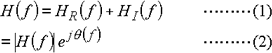|H (f)| represents the amplitude spectrum of the system and θ (f) indicates the phase characteristic of the waveform.

Power Spectrum

The power spectrum is obtained by dividing the signal power into fixed frequency bands and expressing the power of each band as a function of frequency. The unit is the square of the amplitude (V2rms).

With the FFT analyzer, the Fourier transform pair for time function x (t) is represented by the following expression: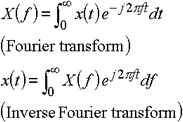The complex function X (f) is a Fourier spectrum of time function x (t). As shown above, if the Fourier spectrum is known, the original time function can be obtained.

Actually, in order to perform numerical calculation from sample values with limited length, Discrete Fourier transform is performed. With the FFT analyzer, Fast Fourier Transform is used which is the high-speed operation of the DFT.

Although the dimension of the power spectrum is (V2 rms), this FFT analyzer uses √(V2 rms) for the linear scale, which agrees with the execution value of the time waveform of the frequency. Display of (V2 rms) is also possible using a menu.

With the default setting, the X axis is assigned the frequency and the Y axis is assigned a logarithmic scale where 1 V2 rms corresponds to 0 dBV rms.

Power spectrum (Linear/Log conversion)

Enter a half-width number in the box below and press the original unit button. The converted value in each units are displayed in the lower box.

V rms : RMS value of power spectrum displayed in linear
V 0-p : Zero to Peak value of power spectrum displayed in linear
dBVr : RMS value of power spectrum displayed in log
dBV0-p : Zero to Peak value of power spectrum displayed in log

The conversion is calculated as

V 0-p = √2×V rms

dBVr = 10 LOG{Vrms /(reference)}2 = 20 LOG (Vrms) [The reference is the rms value 1 Vrms.]

The calculated value may not exactly match the true value due to the effective digits.

Power Spectrum Density

With the FFT analyzer, the bandwidth, Δf, depends on the frequency range. When the resolution is 1/800, for example, the bandwidth equals 20kHz/800=25Hz in the 20kHz range.

When frequency analysis is performed for white noise or other signals distributed over a wide range, the power is obtained as an integral value for each bandwidth. Therefore, if the frequency range is changed, the power also changes, disabling comparison. Then, the following method can be used to obtain the power spectrum for the unit frequency (1 Hz), which, however, is meaningless for line spectrum signals.

The power spectrum density is calculated as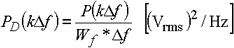where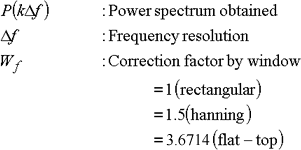The power obtained in the bandwidth corresponding to each window is standardized. When calculating the power spectrum density, perform measurement, if possible, using the hanning window or rectangular window.